Updating search results...

# 7 Results

View
Selected filters:
• UT.MATH.III.SIII.MP.4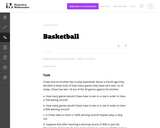Unrestricted Use
CC BY
Rating
0.0 stars

This task provides a simple but interesting and realistic context in which students are led to set up a rational equation (and a rational inequality) in one variable, and then solve that equation/inequality for an unknown variable.

Subject:
Mathematics
Secondary Mathematics
Material Type:
Activity/Lab
Provider:
Illustrative Mathematics
Provider Set:
Illustrative Mathematics
Author:
Illustrative Mathematics
12/15/2012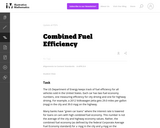Unrestricted Use
CC BY
Rating
0.0 stars

The primary purpose of this problem is to rewrite simple rational expressions in different forms to exhibit different aspects of the expression, in the context of a relevant real-world context (the fuel efficiency of of a car). Indeed, the given form of the combined fuel economy computation is useful for direct calculation, but if asked for an approximation, is not particularly helpful.

Subject:
Mathematics
Secondary Mathematics
Material Type:
Activity/Lab
Provider:
Illustrative Mathematics
Provider Set:
Illustrative Mathematics
Author:
Illustrative Mathematics
01/25/2013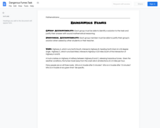Restricted Use
Rating
0.0 stars

Students will work to solve a problem involving a 30-60-90 right triangle and a constant rate of change to discover how long it will take for dangerous fumes from a car crash to each others on the road.

Subject:
Mathematics
Material Type:
Activity/Lab
Provider:
Complex Instruction Consortium
10/24/2022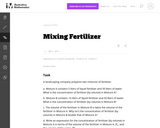Unrestricted Use
CC BY
Rating
0.0 stars

The problem deals with a rational expression which is built up from operations arising naturally in a context: adding the volumes of the fertilizer and the water, and dividing the volume of the fertilizer by the resulting sum. Thus it encourages students to see the expression as having meaning in terms of numbers and operations, rather than as an abstract arrangement of symbols.

Subject:
Mathematics
Secondary Mathematics
Material Type:
Activity/Lab
Provider:
Illustrative Mathematics
Provider Set:
Illustrative Mathematics
Author:
Illustrative Mathematics
05/01/2012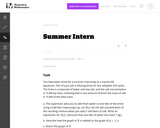Unrestricted Use
CC BY
Rating
0.0 stars

In this task students must figure out how much fresh water you must add to get a particular salt concentration in aquarium water.

Subject:
Mathematics
Secondary Mathematics
Material Type:
Activity/Lab
Provider:
Illustrative Mathematics
Provider Set:
Illustrative Mathematics
Author:
Illustrative Mathematics
05/01/2012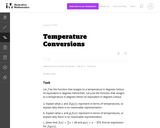Unrestricted Use
CC BY
Rating
0.0 stars

These unit conversion problems provide a rich source of examples both for composition of functions (when several successive conversions are required) and inverses (units can always be converted in either of two directions).

Subject:
Mathematics
Secondary Mathematics
Material Type:
Activity/Lab
Provider:
Illustrative Mathematics
Provider Set:
Illustrative Mathematics
Author:
Illustrative Mathematics
05/01/2012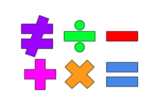Unrestricted Use
Public Domain
Rating
0.0 stars

This lesson is for a math classroom, but can be adapted to fit any grade, subject, or content.&nbsp;&nbsp;In this lesson, students will use an iPad and its features: Keynote, Pages, Garageband, Numbers, Presentation, and iMovie.&nbsp; Students will use 3 of those features/programs to create a video lesson consisting of several examples from a topic of their choice.&nbsp; This project is in place of a term final, so their chosen topic should be from their current term.Image citation: The image is one I created.&nbsp;&nbsp;

Subject:
Secondary Mathematics
Material Type:
Assessment
Author:
Tyrani Bevell